# Texas Go Math Grade 1 Lesson 5.6 Answer Key Take Apart Numbers

Refer to our Texas Go Math Grade 1 Answer Key Pdf to score good marks in the exams. Test yourself by practicing the problems from Texas Go Math Grade 1 Lesson 5.6 Answer Key Take Apart Numbers.

## Texas Go Math Grade 1 Lesson 5.6 Answer Key Take Apart Numbers

Essential Question
How can you show ways to take apart a number?
Explanation:
We have to write partners for the number
4 partners are 1 and 3, 2 and 2, 3 and 1

Explore
Use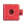to show all the ways to take apart 5.
Color and draw to show your work.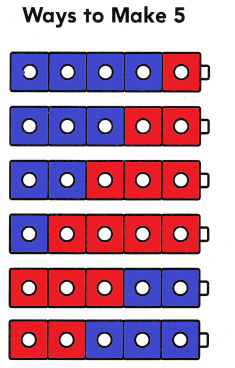Explanation:
The above picture shows how Jenny  shares the pennies
the ways to make 5 flowers

For The Teacher

• Read the following problem, and have children show all the ways to solve the problem. Jenny has S pennies. What are all the ways she can group the pennies?
Explanation:
5 – 4 = 1
5 – 3 = 2
5 – 2 = 1
5 – 1 = 4

Math Talk
Mathematical Processes
How do you know you showed all the ways? Explain.
Explanation:
The process of numbers will be repeated
after once it is completed.

Model and Draw

Now Jenny has 9 pennies. What are all the ways she can group the pennies?

Complete the number sentence.

Question 1.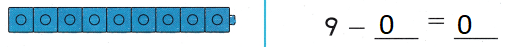Explanation
9 – 0 = 9
Jenny has 9 pennies now

Question 2.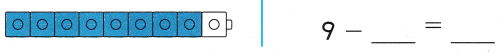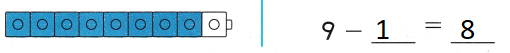Explanation
9 – 1 = 8
Jenny has 8 pennies now

Share and Show

UseColor and draw to show how to take apart 9. Complete the subtraction sentence.

Question 3.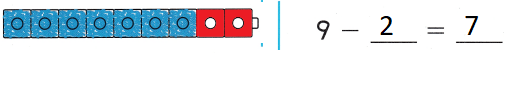Explanation
The pennies that Jenny have is 9 – 2 = 7

Question 4.Explanation
The pennies that Jenny have is 6
9 – 3 = 6

Question 5.Explanation
The pennies that Jenny have is 5
9 – 4 = 5

Question 6.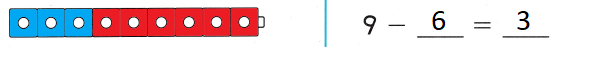Explanation
The pennies that Jenny have is 3
9 – 6 = 3

Question 7.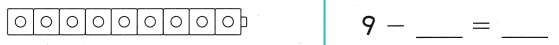Explanation
The pennies that Jenny have is 2
9 – 7 = 2

Question 8.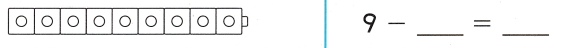Explanation
The pennies that Jenny have is 1
9 – 8 = 1

Question 9.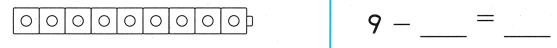Explanation
9 – 0 = 9
Jenny has 9 pennies now

Question 10.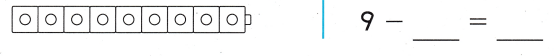Explanation
The pennies that Jenny have is 4
9 – 5 = 4

Problem Solving
Use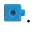Color and draw to show ways to take apart 10. Complete the subtraction sentence.

Question 11.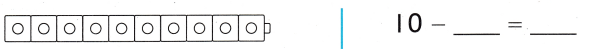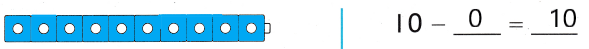Explanation:
10 – 0 = 0
The number subtracted with self the difference will be it self

Question 12.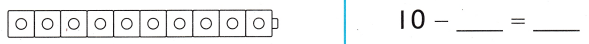Explanation:
The difference of 10 and 2 = 8
10 – 2 = 8

Question 13.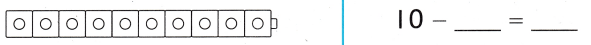Explanation:
The difference of 10 and 3 = 7
10 – 3 = 7

Question 14.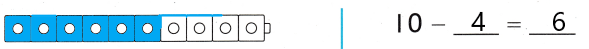Explanation:
The difference of 10 and 4 = 6
10 – 4 = 6

Write or draw to solve.

Question 15.
Hannah has 7 shells. She shares them with Emily. Draw two ways she can share the shells.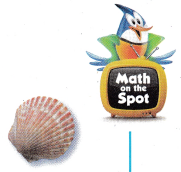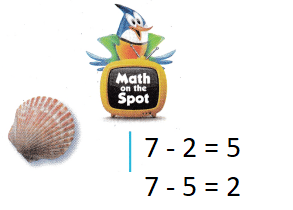Explanation:
Hannah has 7 shells.
She shares them with Emily.
7 – 2 = 5
7 – 5 = 2

Question 16.
H.O.T. Multi-Step Marta has 7 tickets. She shares them with Pete. She shares fewer than 5 tickets. Complete two ways she can share them.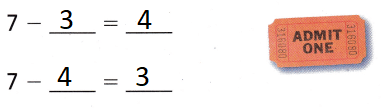Explanation:
Marta has 7 tickets. She shares them with Pete.
She shares fewer than 5 tickets.
Fewer than 5 is 4 and 3
7 – 4 = 3
7 – 3 = 4

Question 17.
There are 8 puffins skating. Then 3 puffins stop to rest. Which shows how many puffins are still skating?(A) 11 – 8 = 3
(B) 8 – 3 = 5
(C) 8 + 3 = 11
Explanation:
There are 8 puffins skating. Then 3 puffins stop to rest.
8 – 3 = 5
5 puffins are still skating

Question 18.
Mathew and Penny have 7 peanuts. Which shows a way they can share the peanuts?
(A) 7 – 4 = 3
(B) 10 – 3 = 7
(C) 7 + 7 = 14
Explanation:
7 – 4 = 3
shows a way  that they can share the peanuts

Question 19.
Use Tools Anne has 6 dolls. She gives 1 doll to her sister. Then she gives 1 more doll to her sister. How many dolls does Anne have now?(A) 4
(B) 2
(C) 3
Explanation:
1 + 1 = 2
6 – 2 = 4
4 dolls that Anne have now

Question 20.
Texas Test Prep Which shows a way to take apart 9?
(A) 10 – 9 = 1
(B) 9 + 9 = 18
(C) 9 – 1 = 8
Explanation:
10 – 9 = 1
shows the way to take apart 9

Take Home Activity

• Write 5 – 0 = 5 and 5 – 1 = 4. Ask your child to subtract from 5 another way. Take turns to show ways to subtract from 5.
Explanation:5 – 2 = 3
5 – 3 = 2

### Texas Go Math Grade 1 Lesson 5.6 Homework and Practice Answer Key

Color and draw to show ways to take apart 8. Complete the subtraction sentences.

Question 1.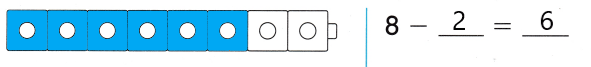Explanation:
The difference of 8 and 2 = 6
8 – 2 = 6

Question 2.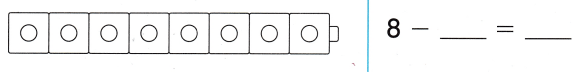Explanation:
The difference of 8 and 3 = 5
8 – 3 = 5

Question 3.Explanation:
The difference of 8 and 4 is 4
8 – 4 = 4

Question 4.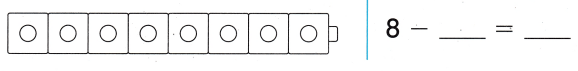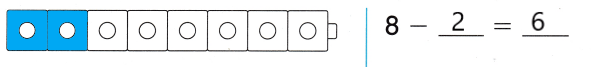Explanation:
The difference of 8 and 2 is 6
8 – 6 = 2

Problem Solving

Question 5.
Bert has 6 apples. He shares them with Carrie. Draw two ways he can share the apples.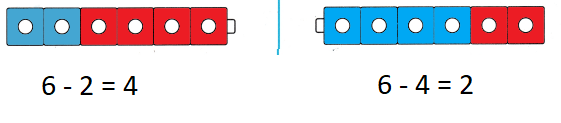Explanation:
6 – 4 = 2
6 – 2 = 4

Texas Test Prep

Lesson Check

Question 6.
5 seals are on the beach. 1 seal goes swimming. Which shows how many seals are still on the beach?
(A) 5 – 1 = 4
(B) 5 + 1 = 6
(C) 6 – 1 = 5Explanation:
5 – 1 = 4
shows that how many seals are still on the beach

Question 7.
Two dogs have 8 bones. Which shows a way they can share the bones?
(A) 10 – 2 = 8
(B) 8 + 1 = 9
(C) 8 – 4 = 4
Explanation:
10 – 2 = 8
shows a way that they can share the bones

Question 8.
Multi-Step Lin has 10 pennies. She shares them with Paul. She shares more than 6 pennies. Which number sentence shows a way she could share the pennies?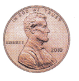(A) 10 – 6 = 4
(B) 10 – 8 = 2
(C) 10 – 1 = 9
Explanation:
Lin has 10 pennies. She shares them with Paul.
She shares more than 6 pennies.
More than 6 is 10 – 8 = 2

Question 9.
Which shows a way to take apart 6?
(A) 6 – 5 = 1
(B) 10 – 6 = 4
(C) 6 + 1 = 7